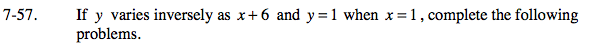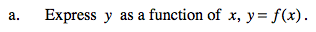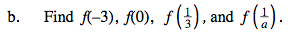### Home > PC > Chapter 7 > Lesson 7.2.2 > Problem7-57

7-57.$\text{If }y\text{ varies inversely as }x, \text{ then }y=\frac{k}{x}.$

$y=\frac{k}{x+6}$

Use the fact that y = 1 when x = 1 to solve for k.

Substitute k back into your original equation.
To write y as a function of x, change y = to f(x) =.$f\left ( \frac{1}{a} \right )=\frac{7a}{1+6a}$